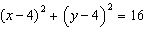Name:    Geometry Chapter 10 - Circles

Multiple Choice
Identify the choice that best completes the statement or answers the question.

1.

Identify two chords.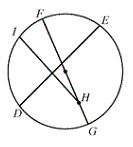a.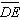and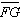c.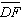and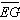b.and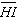d.and2.

Given: In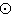O, m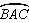= 300. Find m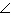A.a. 30c. 18b. 15d. 363.

How many degrees does a minute hand move in 5 minutes?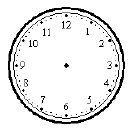a. 30c. 20b. 25d. 504.

A minor arc ofP is _____.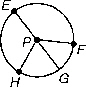a.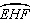c.b.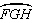d.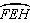Use the diagram (not drawn to scale) and the given information.5.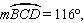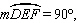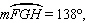and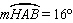Find m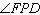.
 a. 24c. 16b. 100d. 376.

m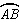= 82°, m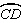= 30°
Find mDOC.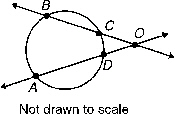a. 112° c. 26° b. 56° d. 52°

Use the diagram (not draw to scale) and the given information.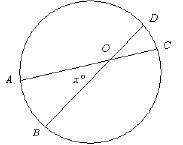7.

Find the value of x if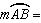20° and62°.
 a. 41° c. 43° b. 21° d. 20.5°

Find the value of x.

8.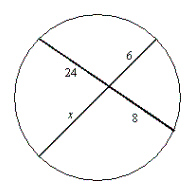a. 12 c. 6 b. 16 d. 32

9.

Write the standard equation of a circle with its center at the origin and radius 7.
 a.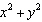= 49 c.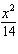+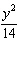= 1 b.= 14 d.= 7

10.

Write the standard equation of a circle with center (4, –4) and radius 4.
 a.c.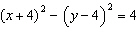b.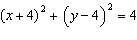d.